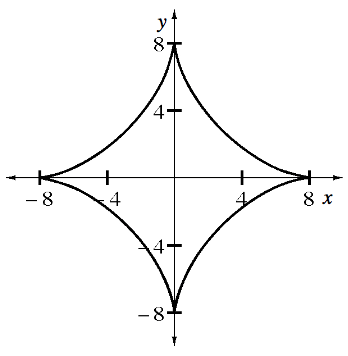### Home > APCALC > Chapter 6 > Lesson 6.2.2 > Problem6-72

6-72.

ASTROID

The graph of $x^{2/3}+y^{2/3}=4$ is called an astroid. .

1. Use implicit differentiation to find $\frac { d y } { d x }$.

$\frac{2}{3}x^{-1/3}+\frac{2}{3}y^{-1/3}y^\prime=0$

$\frac{2}{3}x^{-1/3}=-\frac{2}{3}y^{-1/3}y^\prime$

$y^\prime=-\frac{y^{1/3}}{x^{1/3}}$

$\frac{dy}{dx}=-\sqrt{\frac{y}{x}}$

2. Where does the astroid have a horizontal tangent? Explain.

Look at the derivative equation. Will values of $y$ or values of $x$ make the derivative $0$?

A horizontal tangent should exist when $\frac{dy}{dx}=0$, which happens when $y=0$.

Now look at the graph. There are two coordinate points in which $y=0$. Does the derivative exist at those points?
Now look at the graph. Describe the shape of the graph at that value of $x$.